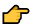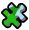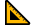# Pythagoras puzzle proof - Classroom

## Start here :

ABC is a right triangle with sides a, b, c as shown.Click on the buttons, they will appear one by one.Then you'll have a puzzle to complete using the three coloured triangles.The triangles can be moved (drag and drop) or rotated using the round yellow handle at the right angles. It's better to go full screen (click at the bottom right) When you get the final green check markanswer the two questions below to prove the pythagorean theorem using this puzzle.
When you get the final green check markanswer the two questions below to prove the pythagorean theorem using this puzzle.
We assume such a puzzle is always possible whatever right triangle ABC we start with. Check how the different lengths and angles fit perfectly together.

Explain why the dashed white line is a mirror line for the final triangle created with this puzzle.

Explain why whatever the right triangle ABC we choose with sides a, b, c where a is the hypotenuse, we have :

=+

## We just proved..

Pythagorean theorem.  In any right triangle, the square of the length of the hypotenuse equals the sum of the squares of the lengths of the legs of the right triangle.